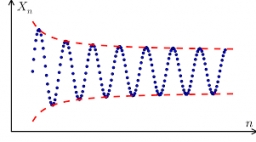# Calculate 22653

In a geometric sequence, the first term is 5, and the quotient is 4.
Calculate the 4th, 6th, and 10th members of this sequence.

a4 =  320
a6 =  5120
a10 =  1310720

### Step-by-step explanation:Did you find an error or inaccuracy? Feel free to write us. Thank you!